Feeds:
Posts

## individual assignment: video

if you want to watch from youtube,

## another poster?

holla..

this is the another poster i’ve made for the assignment. and maybe it’ll be the poster that i’ll assign as 2d assignment.wish me luck on getting high marks..

hoho..

## individual assignment

moshi..moshi!!according to course outlinE TECHNOLOGY IN EDUCATION,

the students should assign a 2d and 3d material that can be use as one of the  teaching tools.

thus, poster is produce as 2d assignment.above is the simple poster i’ve made.

please consider the creativity used and all of you are invited to leave some comments or please do critic it. hee~

thank you..!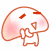## studying xara 3dthis is my studying on xara 3d . its a software on making 3d word.

## studying photoshop

lately, i got the truly deeply home work to study how to edit photo by adobe photoshop. i need to learn how to make a poster using photoshop. this is because i should send a poster assignment which i got from class of Technology in Education. basically, i was already learn how to edit photo by this software, but i am still did not know how to design a poster by using photoshop. this may because of the lack in creativity in myself. fufu~

by the way, in effort on studying how to make a poster, i was finally design a (maybe) poster. but it was about some motivation/advises. any way, take a look at this —->hehe..this wass totally my handwork, also the background.hm..well, i’m still working on it.

wish me luck ok!

## reflection and refraction of light.### Rays and wave fronts

Light is a very complex phenomenon, but in many situations its behavior can be understood with a simple model based on rays and wave fronts. A ray is a thin beam of light that travels in a straight line. A wave front is the line (not necessarily straight) or surface connecting all the light that left a source at the same time. For a source like the Sun, rays radiate out in all directions; the wave fronts are spheres centered on the Sun. If the source is a long way away, the wave fronts can be treated as parallel lines.

Rays and wave fronts can generally be used to represent light when the light is interacting with objects that are much larger than the wavelength of light, which is about 500 nm. In particular, we’ll use rays and wave fronts to analyze how light interacts with mirrors and lenses.

### The law of reflection

Objects can be seen by the light they emit, or, more often, by the light they reflect. Reflected light obeys the law of reflection, that the angle of reflection equals the angle of incidence.For objects such as mirrors, with surfaces so smooth that any hills or valleys on the surface are smaller than the wavelength of light, the law of reflection applies on a large scale. All the light travelling in one direction and reflecting from the mirror is reflected in one direction; reflection from such objects is known as specular reflection.

Most objects exhibit diffuse reflection, with light being reflected in all directions. All objects obey the law of reflection on a microscopic level, but if the irregularities on the surface of an object are larger than the wavelength of light, which is usually the case, the light reflects off in all directions.

### Plane mirrors

A plane mirror is simply a mirror with a flat surface; all of us use plane mirrors every day, so we’ve got plenty of experience with them. Images produced by plane mirrors have a number of properties, including:

1. the image produced is upright
2. the image is the same size as the object (i.e., the magnification is m = 1)
3. the image is the same distance from the mirror as the object appears to be (i.e., the image distance = the object distance)
4. the image is a virtual image, as opposed to a real image, because the light rays do not actually pass through the image. This also implies that an image could not be focused on a screen placed at the location where the image is.

### A little geometry

Dealing with light in terms of rays is known as geometrical optics, for good reason: there is a lot of geometry involved. It’s relatively straight-forward geometry, all based on similar triangles, but we should review that for a plane mirror.

Consider an object placed a certain distance in front of a mirror, as shown in the diagram. To figure out where the image of this object is located, a ray diagram can be used. In a ray diagram, rays of light are drawn from the object to the mirror, along with the rays that reflect off the mirror. The image will be found where the reflected rays intersect. Note that the reflected rays obey the law of reflection. What you notice is that the reflected rays diverge from the mirror; they must be extended back to find the place where they intersect, and that’s where the image is.Analyzing this a little further, it’s easy to see that the height of the image is the same as the height of the object. Using the similar triangles ABC and EDC, it can also be seen that the distance from the object to the mirror is the same as the distance from the image to the mirror.

### Spherical mirrors

Light reflecting off a flat mirror is one thing, but what happens when light reflects off a curved surface? We’ll take a look at what happens when light reflects from a spherical mirror, because it turns out that, using reasonable approximations, this analysis is fairly straight-forward. The image you see is located either where the reflected light converges, or where the reflected light appears to diverge from.

A spherical mirror is simply a piece cut out of a reflective sphere. It has a center of curvature, C, which corresponds to the center of the sphere it was cut from; a radius of curvature, R, which corresponds to the radius of the sphere; and a focal point (the point where parallel light rays are focused to) which is located half the distance from the mirror to the center of curvature. The focal length, f, is therefore:

focal length of a spherical mirror : f = R / 2

This is actually an approximation. Parabolic mirrors are really the only mirrors that focus parallel rays to a single focal point, but as long as the rays don’t get too far from the principal axis then the equation above applies for spherical mirrors. The diagram shows the principal axis, focal point (F), and center of curvature for both a concave and convex spherical mirror.Spherical mirrors are either concave (converging) mirrors or convex (diverging) mirrors, depending on which side of the spherical surface is reflective. If the inside surface is reflective, the mirror is concave; if the outside is reflective, it’s a convex mirror. Concave mirrors can form either real or virtual images, depending on where the object is relative to the focal point. A convex mirror can only form virtual images. A real image is an image that the light rays from the object actually pass through; a virtual image is formed because the light rays can be extended back to meet at the image position, but they don’t actually go through the image position.

### Ray diagrams

To determine where the image is, it is very helpful to draw a ray diagram. The image will be located at the place where the rays intersect. You could just draw random rays from the object to the mirror and follow the reflected rays, but there are three rays in particular that are very easy to draw.

Only two rays are necessary to locate the image on a ray diagram, but it’s useful to add the third as a check. The first is the parallel ray; it is drawn from the tip of the object parallel to the principal axis. It then reflects off the mirror and either passes through the focal point, or can be extended back to pass through the focal point.

The second ray is the chief ray. This is drawn from the tip of the object to the mirror through the center of curvature. This ray will hit the mirror at a 90° angle, reflecting back the way it came. The chief and parallel rays meet at the tip of the image.

The third ray, the focal ray, is a mirror image of the parallel ray. The focal ray is drawn from the tip of the object through (or towards) the focal point, reflecting off the mirror parallel to the principal axis. All three rays should meet at the same point.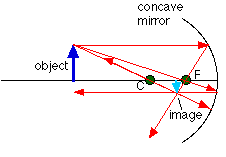A ray diagram for a concave mirror is shown above. This shows a few different things. For this object, located beyond the center of curvature from the mirror, the image lies between the focal point (F) and the center of curvature. The image is inverted compared to the object, and it is also a real image, because the light rays actually pass through the point where the image is located.

With a concave mirror, any object beyond C will always have an image that is real, inverted compared to the object, and between F and C. You can always trade the object and image places (that just reverses all the arrows on the ray diagram), so any object placed between F and C will have an image that is real, inverted, and beyond C. What happens when the object is between F and the mirror? You should draw the ray diagram to convince yourself that the image will be behind the mirror, making it a virtual image, and it will be upright compared to the object.

### A ray diagram for a convex mirror

What happens with a convex mirror? In this case the ray diagram looks like this:As the ray diagram shows, the image for a convex mirror is virtual, and upright compared to the object. A convex mirror is the kind of mirror used for security in stores, and is also the kind of mirror used on the passenger side of many cars (“Objects in mirror are closer than they appear.”). A convex mirror will reflect a set of parallel rays in all directions; conversely, it will also take light from all directions and reflect it in one direction, which is exactly how it’s used in stores and cars.

### The mirror equation

Drawing a ray diagram is a great way to get a rough idea of how big the image of an object is, and where the image is located. We can also calculate these things precisely, using something known as the mirror equation. The textbook does a nice job of deriving this equation in section 25.6, using the geometry of similar triangles.### Magnification

In most cases the height of the image differs from the height of the object, meaning that the mirror has done some magnifying (or reducing). The magnification, m, is defined as the ratio of the image height to the object height, which is closely related to the ratio of the image distance to the object distance: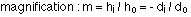A magnification of 1 (plus or minus) means that the image is the same size as the object. If m has a magnitude greater than 1 the image is larger than the object, and an m with a magnitude less than 1 means the image is smaller than the object. If the magnification is positive, the image is upright compared to the object; if m is negative, the image is inverted compared to the object.

### Sign conventions

What does a positive or negative image height or image distance mean? To figure out what the signs mean, take the side of the mirror where the object is to be the positive side. Any distances measured on that side are positive. Distances measured on the other side are negative.

f, the focal length, is positive for a concave mirror, and negative for a convex mirror.

When the image distance is positive, the image is on the same side of the mirror as the object, and it is real and inverted. When the image distance is negative, the image is behind the mirror, so the image is virtual and upright.

A negative m means that the image is inverted. Positive means an upright image.

### Steps for analyzing mirror problems

There are basically three steps to follow to analyze any mirror problem, which generally means determining where the image of an object is located, and determining what kind of image it is (real or virtual, upright or inverted).

• Step 1 – Draw a ray diagram. The more careful you are in constructing this, the better idea you’ll have of where the image is.
• Step 2 – Apply the mirror equation to determine the image distance. (Or to find the object distance, or the focal length, depending on what is given.)
• Step 3 – Make sure steps 1 and 2 are consistent with each other.

### Refraction

When we talk about the speed of light, we’re usually talking about the speed of light in a vacuum, which is 3.00 x 108 m/s. When light travels through something else, such as glass, diamond, or plastic, it travels at a different speed. The speed of light in a given material is related to a quantity called the index of refraction, n, which is defined as the ratio of the speed of light in vacuum to the speed of light in the medium:

index of refraction : n = c / v

When light travels from one medium to another, the speed changes, as does the wavelength. The index of refraction can also be stated in terms of wavelength:Although the speed changes and wavelength changes, the frequency of the light will be constant. The frequency, wavelength, and speed are related by: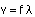The change in speed that occurs when light passes from one medium to another is responsible for the bending of light, or refraction, that takes place at an interface. If light is travelling from medium 1 into medium 2, and angles are measured from the normal to the interface, the angle of transmission of the light into the second medium is related to the angle of incidence by Snell’s law :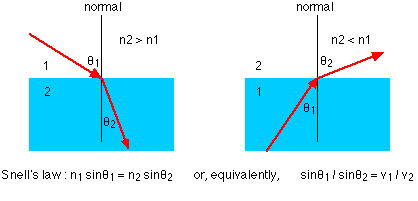Reflection & refraction of light

this is the power point short note about the reflection and refraction of light.

## Nasihat Untuk-mu : HATI

Berkata- kata Si sang bijaksana, sucinya hati seseorang maka terpancarlah hikmah dilidahnya”. Makin banyak bicara, makin banyak pula kesalahannya. mukmin itu diamnya fikir..bicaranya zikir”.

Dan engkau perangkan nafsu dengan perlaparkan dia dan jaga dia”

Kata-kata hikmah ini terbit takkala mengingati kisah para sahabat Rasulullah s.a.w. baru sahaja pulang dari berperang dengan musuh Islam. Baginda menyatakan, “ini baru sahaja jihad yang kecil, sebaliknya jihad yang paling besar adalah memerangi hawa nafsu”

Ayat ini juga memberi solusi kepada kita bagaimana untuk berperang dengan hawa nafsu. Ini di ambil dari kisah angkuhnya nafsu kepada Allah s.w.t. …. (see more)

## happy chinese new year !i want to wish “HAPPY CHINESE NEW YEAR”

to all chinese. may this new year will be better than the last year.

Gong xi Fa Chai!!

## basic physicswhat is PHYSICS?

Physics is the scientific study of matter and energy and how they interact with each other.

This energy can take the form of motion, light, electricity, radiation, gravity . . . just about anything, honestly. Physics deals with matter on scales ranging from sub-atomic particles (i.e. the particles that make up the atom and the particles that make up those particles) to stars and even entire galaxies……………(more)